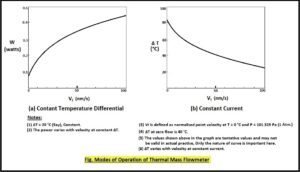# Direct Mass Flow Measurement

## Mass Flow Meters with no moving parts

In this series, we shall see the principles of operation of various types of flow meters. First, we will discuss the principles of different types of Mass Flow Meters and later the principles on which the volumetric flow meters are based.

Direct Mass Flow Meters:
The meters, where the principle used is based on direct mass flow measurement i.e. when the signal generated is directly related to mass flow rate, are called Direct Mass Flow Meters.
We will discuss one type of Direct Mass Flow Measurement principle here.

Thermal Dispersion Mass Flow Meters:
Whenever a fluid of a certain temperature is passed over any substance hotter than the fluid, the heat of the hot substance is taken away because of forced convection by the fluid, and the temperature of that substance drops.
This fall in temperature is related to the mass flow of the fluid passing over the substance. If one can measure the amount of heat taken away by the flowing fluid one can calculate the mass flow rate.

In another way, if one can maintain the temperature difference between the flowing fluid and the tiny heater constantly by additional heat to the heater, the measurement of additional heat is having a direct relation with Mass Flow Rate. This will be a Direct Mass Flow measurement.

The additional power required to heat the heater has a little complicated relationship with the mass flow rate.

This was discovered by L.V. King in 1914. He stated this fact through his famous King’s Law.

A heated wire can measure the mass velocity at a point when immersed in a flowing fluid.

The technic is called Hot Wire Anemometry. In around 1970, the industrial-grade Thermal Dispersion Mass Flow Meters were available.

King’s Law states,
W = W0 + K(Qm)n
Where,
W: Heater Power during the flowing conditions.
W0: Heater Power at no-flow condition.
K: Constant, Device dependent.
Qm: Mass Flow rate.
n: Dimensionless figure (typically between 0.25 to 0.5)

Types of Measuring Methods:
There are two types of measurement methods. In both methods, heat is taken away from the heater by the flowing fluid.

• Thermal dispersion or immersible type,
• Capillary type Thermal Mass Flow Meter.

In the case of Thermal dispersion or immersible type, the heat is transferred to the layer of the flowing fluid coming in contact with the heater surface. The convected heat from the surface of the heating element is related to the point mass velocity of the fluid.

In the second type, i.e. capillary type thermal mass flow meter, the heat is transferred to the bulk of fluid passing through the small heated capillary tube.
Although the principles of operation of both types depend on heat convicted, both methods are so different that two separate articles are required to explain the same. This Article talks about the principle of Thermal Dispersion immiscible type mass flow meter and its applications in industries.

Modes of Thermal Dispersion Mass Flow Meters :
There are two modes of measurement methods,

• Constant Temperature Differential mode,
• Constant Current mode.

Let us see what are these two modes of measurement.

• In Constant Temperature operation mode, the heater probe usually called a velocity probe or velocity sensor is always kept at some fixed temperature that is higher than the fluid temperature, say 200C. When the fluid passes over the heated surface of the velocity sensor it takes away some heat thereby dropping the temperature of the velocity sensor. If we refill the heat so as to obtain the same temperature differential then this heat required is a measure of fluid point mass velocity.
ΔT = Th – T∞ Where,
Th is the temperature of velocity sensor,
T∞ is the temperature of the flowing fluid.
In this case, the output is the power supplied to the velocity sensor to maintain the ΔT.
The relation is given above as King’s Law.
• In the Constant Current mode of operation, the heating current supplied to the velocity sensor is kept constant. In this case, the ΔT varies with the flow and is the output signal as a measure of flow rate.

The basic reasons for the popularity of Thermal Mass Flow Meters :

• Simple for installation.
• There are no moving parts in the flow meter.
• Pressure and Temperature compensations are not required.
• Almost unobstructed straight flow path.
• Very negligible pressure loss and pressure requirement, unlike differential pressure flow meters.
• Straight pipe runs can be reduced by using flow conditioners or flow straighteners.
• Very good repeat accuracy.

Industrial Applications :

• Compressed Air / Gas consumption measurement.
• Very low pressure flowing conditions.
• Fuel gases consumption measurement.
• Furnace and burner control.
• Biogas measurement at digester and ahead.
• HVAC Applications.

……. And many more.
In the next article, we will discuss the principle of the Coriolis Mass Flow Meter.#### 1 Comment

•Nitin

I am interested in your mass flow meters